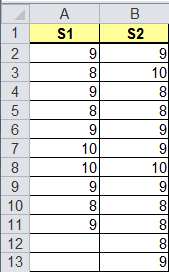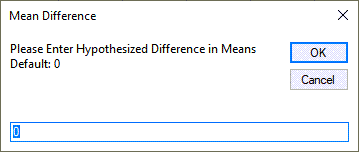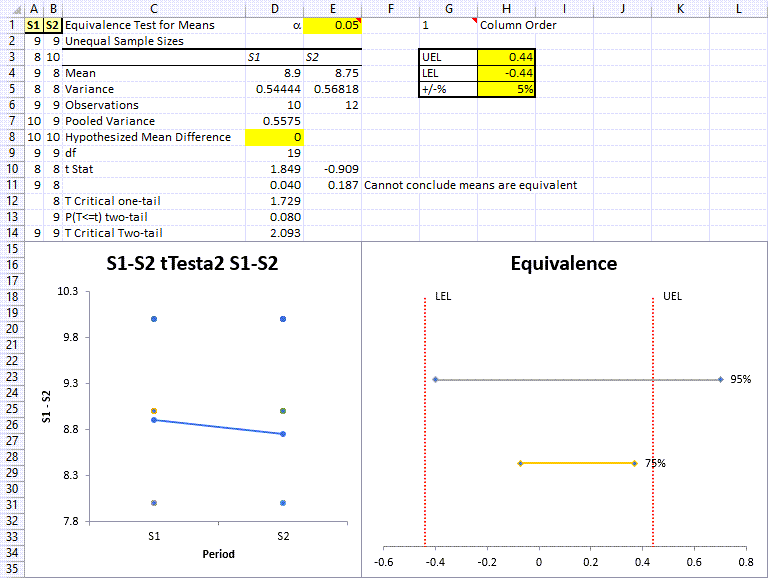# Want a Two One-Sided Equivalence Test (TOST) in Excel?

## QI Macros can do it for you!

### Go Deeper: When to use an Equivalence Test

Traditional t-Tests determine if means are the same or different, but they can have false positives. Equivalence testing determines an interval where the means can be considered equivalent.

The Equivalence (TOST) Test uses two t-tests assuming equal variances with a hypothesized mean difference (u1-u2 = interval).

Note: Excel does not do equivalence tests; QI Macros provides this functionality.

### Equivalence Test Example

Adhesive tape is measured immediately after production and 24 hours later. Are the measurements equivalent at these two different points in time? If they are, one measurement can be eliminated, saving time and money.#### Run an Equivalence (TOST) Test Using QI Macros

Select the data within your Excel spreadsheet, click on the QI Macros menu > Statistical Tools > F and t Tests and select Equivalence (TOST) Test:QI Macros will prompt for a significance level (default = 0.05 or 0.95):Along with the hypothesized mean difference (Note: This difference will set the range acceptable for equivalence. Default = 0):Calculation used:NOTE: The Hypothesized Mean Difference is the hypothesized difference between the means in your data set. The HMD informs the t Stat calculation, which then informs the p value. If you have a calculated HMD that is much greater than the actual difference between your two means, it will dramatically affect your p value.

The Equivalence test will perform the calculations and interpret the results for you!
If both p-values are less than 0.05, the means are equivalent.Included in the July 2022 release, we provide a Values Plot along with an Equivalence Plot.

All releases prior to July 2022 included a Box & Whisker output only.

#### Now here's where it gets interesting...because the one-tailed p-values are both less than 0.05...the means can be declared equivalent. So, we can eliminate one of the tests.

Define the two null and alternate Hypotheses for equivalence:

• The null hypothesis H01 is that the mean difference (x1-x2) <= 0.8
• The alternative hypothesis H11a is that the mean difference > 0.8
• The null hypothesis H02 is that the mean difference (x1-x2) >= 0.8
• The alternative hypothesis H12a is that the mean difference < 0.8

### Equivalence Plot

UEL (Upper Equivalence Limit ‐ upper limit of acceptability for the difference) and LEL (Lower Equivalence Limit ‐ lower limit of acceptability for the difference) are based off the sum of the 2 data sets' means, divided by 2, and multiplied by the +/‐% value found in cell H5 (this value affects the width of UEL/LEL).

NOTE: What is in between UEL and LEL is considered to be the "Zone of Equivalence."

The UEL, LEL, and Confidence Interval CAN BE UPDATED BY THE END USER in cells H3:H5 accordingly. And if the UEL and LEL are updated, the Confidence Interval lines and Equivalent/Not Equivalent statements will update automatically, based upon your data set.

95% line output represents your 95% Confidence Interval and since it is partially located outside of the Zone of Equivalence, this indicates that your data set statistically CANNOT claim equivalence.

75% line output represents your 75% Confidence Interval. And per information provided above, since it IS located w/in the Zone of Equivalence, if the end user chooses to use only a 75% Level of Confidence benchmark, their data set CAN statistically claim equivalence

### Why Choose QI Macros Statistical Software for Excel?### Easy to Use

• Works Right in Excel
• Interprets p-values for You
• Accurate No-Worry Results
• Free Training Anytime### Proven and Trusted

• 100,000 Users in 80 Countries
• Celebrating 20th Anniversary
• Five Star CNET Rating - Virus Free### Affordable

• Only \$349 USD
Quantity Discounts Available
• No annual fees
• Free Technical Support